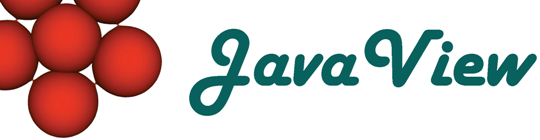Overview  Animation  Classic Surfaces  Parm Surfaces  Curves on Surfaces  Discrete Geodesics  ODE  Platonic Solids  Cycloid  Root Finder  Harmonic Maps  Rivara Bisection  Scalar Field  Weierstrass  Closed Polygon  Elastic Curve  Billiard in an Ellipse  LIC Visualization  Discrete VF  Hodge Splitting  Textured Surface  Surfaces of Rotation  Mean Curvature Flow  Pythagoraen Tree  Julia Sets

## Hodge Splitting of Vector Fields on Surfaces

Study Hodge splitting of discrete vector fields. A vector field is split in a rotation free part (gradU) that has divergence, a divergence free part (CoGradW) that has rotation and a remaining harmonic component. A potential is calculated for each of the rotation free (u) and divergence free (w) parts. The rotation free vector field is the gradient of its potential u and the divergence free vector field is the 90 degree rotated gradient of the potential w.

Hodge Splitting of Vectorfields

In this example a potential creates the original vector field that is then decomposed. The centers of the potential are the vertices of a point set. Keep pressing key 'a' while clicking the left mouse button to add a vertex of type as specified at 'Vertex Type'. Click and drag single vertices to move them around and see the decomposition follow your changes.

You may also invoke a LIC image generator that computes Line Integral Convolution images for the original and all decomposed vector fields. Press the button 'Compute LIC Image' to do that.

Description of the other panels:

 Domain Change dimensions and discretization of the triangulation. Min U Panel that controls the minimization process that is used to generate the potential of the rotation free component. Min W Panel that controls the minimization process that is used to generate the potential of the divergence free component.